# Elliptic Function

(redirected from Elliptic functions)
Also found in: Dictionary, Wikipedia.
Related to Elliptic functions: Jacobi's elliptic functions

## elliptic function

[ə′lip·tik ′fəŋk·shən]
(mathematics)
An inverse function of an elliptic integral; alternatively, a doubly periodic, meromorphic function of a complex variable.
McGraw-Hill Dictionary of Scientific & Technical Terms, 6E, Copyright © 2003 by The McGraw-Hill Companies, Inc.
The following article is from The Great Soviet Encyclopedia (1979). It might be outdated or ideologically biased.

## Elliptic Function

any of various functions associated with the inversion of elliptic integrals. Elliptic functions are used in many branches of mathematics and mechanics in both theoretical studies and numerical calculations.

The inverse of the integral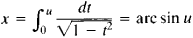is the trigonometric function u = sin x. Similarly, the inversion of normal elliptic integrals of the first kind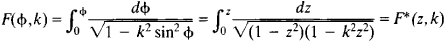(where z = sin φ and k is the modulus of the elliptic integral) gives rise to the functions φ = am z and ω = sn z = sin (am z). The function am z, the amplitude of z, is not an elliptic function.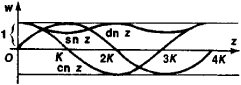Figure 1

The elliptic sine, sn z, is the sine of the amplitude. The functions en z (the cosine of the amplitude) and dn z (the delta of the amplitude) are given by the formulas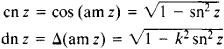The functions sn z, en z, and dn z are called Jacobian elliptic functions. The following relation holds:

sn2z + cn2z = k2 sn2z + dn2z = 1

Figure 1 shows the graphs of the Jacobian elliptic functions for real x and 0 < k < 1;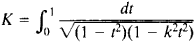is the complete normal elliptic integral of the first kind, and 4K is a primitive period of the elliptic function sn z. In contrast to the singly periodic function sin x, the function sn z is a doubly periodic function. Its second primitive period is 2iK′, where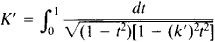and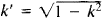is the complementary modulus. The periods, zeros, and poles of the Jacobian elliptic functions are given in Table 1, where m and n are any integers.

Table 1. Properties of Jacobian elliptic functions
FunctionPeriodsZerosPoles
sn z4Km + 2iK′n2mk + 2iK′n2mK + (2n + 1)iK
cn z4K + (2K + 2iK′)n(2m + 1)K + 2iK′n2mK + (2n + 1)ik
dn z2Km + 4iK′n(2m + 1)K + (2n + 1)iK2mK + (2n + 1 )iK

The Weierstrassian elliptic function & (x) may be defined as the inverse of Weierstrass’ normal elliptic integral of the first kind, which has the form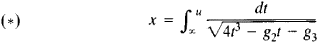where the parameters g2 and g3 are called the invariants of &(x). Here it is assumed that the zeros e1, e2, and e3 of the polynomial 4t3g2tg3 differ from each other; if they did not differ, integral (*) could be expressed in terms of elementary functions. The relations between & (x) and the Jacobian elliptic functions are given by the following equations: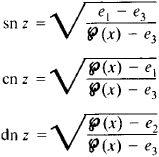Any meromorphic doubly periodic function f(z) is an elliptic function. The ratio of the two periods ω1 and ω2 is imaginary. We thus have f(z + mw1, + nw2) = f(z) whenm m, n = 0, ±1, ±2, . . . and 1m (ω12) ≠ 0. Sigma functions and theta functions are used to construct elliptic functions and to carry out numerical calculations.

The study of elliptic functions was preceded by the investigation of elliptic integrals; a systematic exposition of the theory of such integrals was given by A. Legendre. The founders of the theory of elliptic functions were N. Abel (1827) and K. Jacobi (1829). Jacobi dealt extensively with the theory of the elliptic functions that now bear his name. In 1847, J. Liouville published a discussion of the foundations of the general theory of elliptic functions, considered as meromorphic doubly periodic functions. A representation of elliptic functions in terms of the &-function and the theta and sigma functions was given by K. Weierstrass in the 1840’s (the theta and sigma functions are not elliptic functions).

### REFERENCES

Markushevich, A. I. Teoriia analitkheskikh funklsii, 2nd ed., vol. 2. Moscow, 1968.
Hurwitz, A., and R. Courant. Teoriia funklsii. Moscow, 1968. (Translated from German.)
Whittaker, E. T., and G. N. Watson. Kurs sovremennogo analiza, 2nd ed., part 2. Moscow, 1963. (Translated from English.)
Bateman, H., and A. Erdelyi. Vysshie Iranstsendenlnye funklsii: Ellipticheskie i avtomorfnye funklsii: Funklsii Lame i Mat’e. Moscow, 1967. (Translated from English.)
References in periodicals archive ?
From periodicity of the Jacobi elliptic functions it follows that
Lang, Elliptic Functions, Springer, New York, NY, USA, 2nd edition, 1987
where sn is the Jacobi elliptic function of module s , [tau] is the normalized time, and and are constants specific for the release system.
Various methods have been used to handle nonlinear partial differential equations, such as the Hirota bilinear method , inverse scattering method [2,3] the Backlund transformation method , subequation method [5-7], F-expansion method [8-10], sine-cosine method [11,12], sech-tanh method [13,14], Exp-function method [15,16], and Jacobi elliptic function method [17-19].
where [g.sub.2]([tau]) = 60[G.sub.4]([tau]), [g.sub.3]([tau]) = 140[G.sub.6]([tau]) for the Eisenstein series [G.sub.2k]([tau]) of weight 2k, p(z, [tau]) := p(z, [L.sub.[tau]]) is the Weierstrass elliptic function, and b([tau]), c([tau]) are the coefficients of the Tate normal form contained in [C/[L.sub.[tau]], 1/N + [L.sub.[tau]]].
An alternative approach related to applications of the elliptic functions leads to the crowding phenomenon  that complicates application of the elliptic functions for [delta] < 0.1 as follows from our numeric tests for (2.16).
Nonlinear equation of the beam motion is reduced to linear parametric equation and solved in elliptic functions. The first approximation is harmonic oscillations about some center, elevated above the equilibrium position.
Weierstrass elliptic functions p(z) := p(z,[g.sub.2],[g.sub.3]) have two successive degeneracies and addition formula.
Hazewinkel, Ed., Jacobi Elliptic Functions, Encyclopedia of Mathematics, Springer, New York, NY, USA, 2001.
Although intensive investigations have made significant progress in recent years, many methods have been proposed to construct exact solutions, such as Weierstrass elliptic function method, the homogeneous balance method, sine-cosine method, the nonlinear transformation method, the hyperbolic tangent functions finite expansion, improved mapping approach, and further extended tanh method.
Nevo, "Derivatives of meromorphic functions with multiple zeros and elliptic functions," Acta Mathematica Sinica, vol.
They find that for small times independent of the semiclassical scaling parameter, both types of motion are accurately described by explicit formulae involving elliptic functions, and that these formulae are consistent with predictions of Whitham's formal modulation theory in both the hyperbolic and elliptic cases.

Site: Follow: Share:
Open / Close